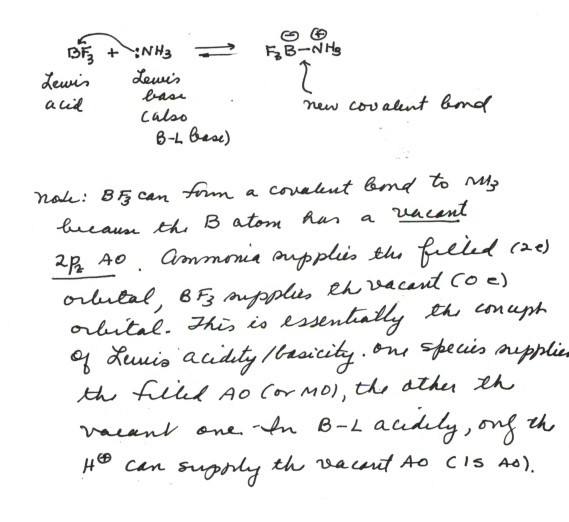TOP

## BRONSTED ACIDS AND BASES IN ORGANIC CHEMISTRY

• You should know that Bronsted-Lowry acids are proton donors, and Bronsted bases are proton acceptors.

• You should be able to write simple Bronsted acid/base equations using curved arrows to show electron flow. The curved arrow should start at an electron pair, either an unshared pair or an electron pair which is involved in a covalent bond.The curved arrow should point to the nucleus (in this case always a proton) to which the electron pair will bind in the product(the right hand side of the equation).

• You should also know that the conjugate base of a Bronsted acid is the species formed when a proton is lost from that acid (e.g., hydroxide ion is the conjugate base of water; water is the conjugate base of the hydronium ion). Similarly, the conjugate acid of a Bronsted base is the species formed when that base is protonated.Thus, water is the conjugate acid of hydroxide ion, and hydronium ion is the conjugate acid of water. In other words, water and hydroxide ion are a conjugate acid/conjugate base pair.

## Quantitative Measurement of Acid and Base Strength

• You should know that the dissociation of a Bronsted-Lowry acid in water produces the conjugate base of the Bronsted acid, along with the hydronium ion. The equilibrium constant K for this dissociation measures the extent of hydronium ion formation and thus, effectively , how strong the Bronsted acid is. In this case strength refers to the relative tendency of the acid to protonate water.

• The acid dissociation constant of the Bronsted-Lowry acid (Ka) is the quantity which is actually used to measure the strength of the acid quantitatively (rather than the equilbrium constant itself). This is simply the product of the hydronium ion concentration and the concentration of the conjugate base of the acid (both on the product side of the equilibrium), divided by the concentration of the Bronsted acid itself(on the left hand side of the equation). Notice that the concentration of water, which is also on the left hand side of the equation, is omitted from the expression, since this is effectively constant in dilute aqueous solution. Thus the acid dissociation constant is the equilibrium constant K multiplied by the constant concentration of water in water.

• Frequently, the pKa, which is the negative common log of the acid dissociation constant is used as a more conveniently-sized number to measure the acidity. Since this is the negative log, a large positive pKa means a large negative exponent of the acid dissociation constant, Ka. this corresponds to a weak acid (Ka greater than 1 indicates more product than reactant; Ka less than 1 indicates more reactant than product; the hydronium ion is on the product side). Conversely, a negative value of the pKa corresponds to a strong acid.

## Consider the following acid dissociation: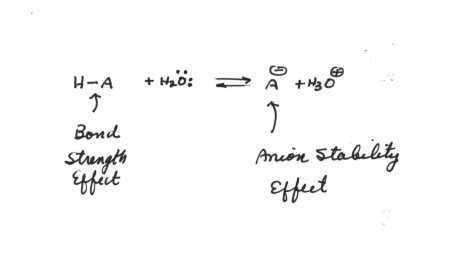The factors which would favor dissociation, i.e., enhance acidity, are:
• A weaker H-A bond, because this destabilizes the left hand(reactant) side of the equation. This is the bond strength affect. A stronger H-A bond tends to decrease the acidity because the bond is harder to break. A weaker H-A bond increases acidity.

• A more stable anion, because this stabilizes the right hand side of the equation. This is the Anion Stability Effect.

### It is an important general rule of thumb that Anion Stability usually exerts the strongest effect upon acidity, i.e., is dominant over bond strength effects. The one special type of exception will be considered shortly.

Anion stability, in turn is affected by (1)resonance stabilization,(2) inductive effects, (3) hybridization effects and (4)electronegativity effects.

We discuss first the exceptional, but important case where bond strength effects predominate.However, even when they are not predominant, it should always be remembered that they always are operative and tend to increase or decrease acidity according to whether the bond is weaker or stronger. If there are opposing anion stability effects, the net effect will usually be qualitatively that predicted by considering the anion stability effect.

## A Case Where Bond Strength Effects Predominate Over Anion Stability Effects

***The Relative Acidities of H-X, i.e., of the hydrogen halides.****

• The pKa's of H-F,H-Cl,H-Br, and H-I are respectively 3.5,-7,-8,and -9. That is, H-F is the weakest acid and H-I the strongest (recall that a negative pKa corresponds to a strong acid.
• Based upon anion stability, the opposite trend should be observed, since fluoride ion should be the most stable of the halide anions (refer to the electronegativity of fluorine, which is the greatest of the halogens.)
• However, the H-F bond is extremely strong and hard to break, so the bond strength effect is acid weakening and is predominant here. The bond dissociation energies of the H-X bonds are, respectively, 136 (HF),103 (HCl),88 (HBr), and 71 (HI). So, the bond strength differences, being large, are greater than the differences in anion stability, causing the former effect to predominate. The effect is largest for fluorine, so that there is relatively little difference in the acidity of the other halogen acids. Thus the two effects are almost, but not quite in balance for these latter three H-X's.
• Similar considerations apply for comparisons in any case involving atoms in the same Group of the Periodic Table. But, rarely are bond strength effects dominant over anion stability effects in any other comparative situation.

## ELECTRONEGATIVITY EFFECTS

Anion stability is strongly affected by the electronegativity of the atom upon which the negative charge rests, i.e., the ability of the atom to stabilize additional electrons.
• For example, negative charge is more stabilized by a fluorine nucleus than a nitrogen nucleus because fluorine has a nucleus which has a positive charge of +9 (nine protons), whereas that of nitrogen has only +7. So pure electrostatic effects(potential energy of attraction of the negatively charged electron to the positively charge nucleus) stabilize electrons on fluorine more than on other atoms. In general, electronegativity is a fairly good neasure of the ability of an atom to stabilize negative charge.
• The electronegativity of fluorine is also greater than that of chlorine , bromine, or iodine, i.e., electronegativity decreases down a column of the Periodic Table. This is because the electron with chlorine, for example, must enter an orbital in the third main shell, which is much further from the nucleus than the second main shell, which would be involved for fluorine. The potential energy of attraction between the electron and the nucleus is inversely proportional to the distance. In the case of a comparison in the same row of the periodic table, the distance factor is the same, because the electrons are entering the same main shell, so it is the charge on the nucleus which is the determining factor of the electronegativity of the atom.
• The pKa's of methane,ammonia,water, and HF are respectively 51, 38, 15.7, and 4.0, i.e., acidity sharply increases upon going from left to right in the periodic Table. This follows the order of anion stability, because the methyl anion is less stable than the amide anion, than the hydroxide anion, than fluoride anion. That is, negative charge is most stable on F, the most electronegative atom, and least stable on carbon, the least electronegative atom of this series. It is true that bond strength effects are in the opposite direction and would tend to make HF the weakest acid, but anion estabilization effects are larger. So it is good to remember that anion stabilization effects are dominant in all cases except when comparisons are made within one group of the periodic table.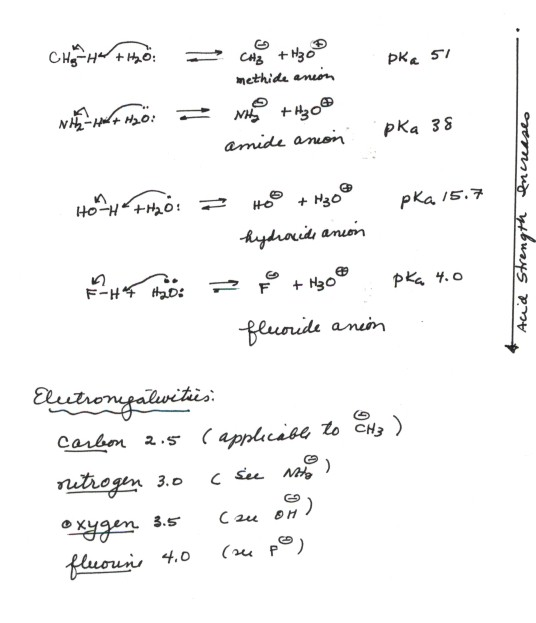## HYBRIDIZATION EFFECTS

***An especially interesting case to consider is the comparison between anions in which the negative charge is on the same atom but the hybridization state of the atom is varied. The most important case here is that of carbon, which, as you know, has three distincet hybridization states.***
• The acidity of ethyne(acetylene) is much greater than than of ethene, which is much greater than than of ethane. The relevant pKa's are 25, 44, and 51.
• The bond strengths would predict the opposite effect, since the C-H bond of ethyne is stronger than that of ethene than that of ethane. So, anion stabilization effects must be operative.
• The relevant anions, as shown in the figure, are the ethynide anion, in which carbon is sp hybridized, the vinyl anion (trigonally or sp2 hybridized), and the ethyl anion (tetrahedrally or sp3 hybridized).The former is the most stable and the last-named one least stable, so that the rule of controlling anion stability is obeyed.
• It is very important to understand the reason for the profound difference in acidity of C-H bonds as a function of their hybridization state.The key point here is that the lower the energy of the orbital in which the unshared pair resides in the anion, the more stable will be the anion. In the ethynide anion, the unshared pair is in a digonal orgabital which is 50%s and 50%p. In the vinyl anion, the unshared pair is in a trigonal orbital which is 33%s and 67%p, while in the ethyl anion the unshared pair is in a tetrahedral orbital which is 25%s and 75%p. Since the energy of an s orbital is much lower than that of the corresponding p orbital, the greater the "% s content" or "s character" of the orbital the lower its energy.Please note that ethyne is almost 20 powers of ten more acidic than ethene.
• It is also important to note that ethyne is 13 powers of ten more acidic than ammonia, even though in the latter case the negative charge in the amide anion is on a nitrogen atom, which has a much higher electronegativeity than carbon. Thus hybridization effects are impressively large, and can cause carbon to appear as even more electronegative than nitrogen, when the former is in the digonal hybridization state.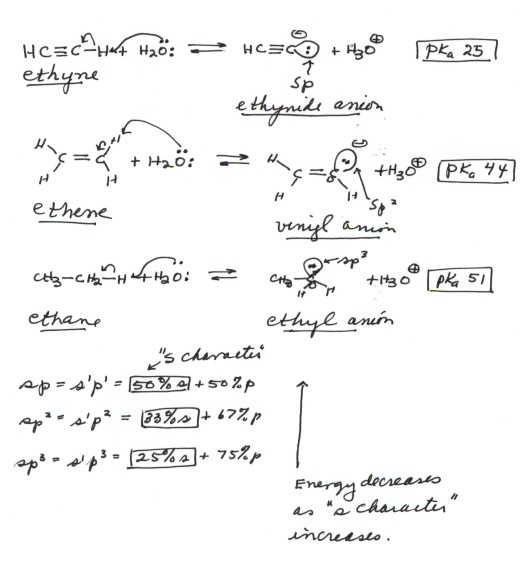## RESONANCE EFFECTS

WE ARE STILL FOCUSING UPON ANION STABILITY AS THE DOMINANT FACTOR IN DETERMINING THE RELATIVE ACIDITIES OF BRONSTED-LOWRY ACIDS. EVEN WHEN THE NEGATIVE CHARGE IS LOCATED ON THE SAME ATOM (SO THAT ELECTRONEGATIVITY IS NOT A FACTOR), AND WHEN THE HYBRIDIZATION STATE OF THE ATOM ISN'T DIFFERENT(SO THAT HYBRIDIZATION EFFECTS ARE NOT IMPORTANT), A SERIES OF ANIONS (CONJUGATE BASES OF THE BRONSTED-LOWRY ACIDS) CAN BE DIFFERENTIALLY STABILIZED BY RESONANCE. AS YOU SHOULD RECALL, DELOCALIZATION OF THE CHARGE OVER MORE THAN ONE ATOM RESULTS IN RESONANCE STABILIZATION. AN EXCELLENT EXMAPLE IS AVAILABLE IN THE COMPARISON OF THE ACIDITIES OF ALCOHOLS(RO-H) WITH CARBOXYLIC ACIDS (RCOOH):

• The conjugate base of an alcohol is an alkoxide anion (RO-). In the case of ethanol, it is ethoxide anion. In such an alkoxide ion, the negative charge is essentially localized upon oxygen. This being an electronegative atom, the negative charge is fairly stable there, so that alcohols are modestly acidic, comparable to water. This is reasonable because in the conjugate base of water, the negative charge is also located upon an oxygen atom.
• The conjugate base of a carboxylic acid is a carboxylate anion. In the case of ,say, acetic acid, it is acetate anion. In such a carboxylate anion, the negative charge is also upon oxygen, but because of resonance effects it is delocalized over two oxygen atoms. Therefore additional stabilization of the anion results, so that carboxylic cacids are typically about 11 powers of ten more acidic than alcohols.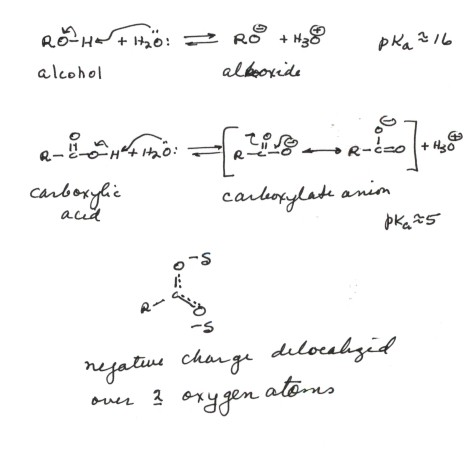## INDUCTIVE EFFECTS

### Note that this section is quite different form the one in our text. For the purposes of this course, please neglect the text discussion and consider the present discussion as replacing it.We are again considering ways in which the anion produced by deprotonating a Bronsted-Lowry acid can be stabilized. The final way which we want to consider is by stabilizing the site of negative charge by interaction with other dipoles which may be present in the molecule. Typically, this kind of effect can be somewhat complex, but the overall result of this stabilization (or destabilization) is referred to as "the inductive effect". As an example, we consider the comparison of acetic acid and chloroacetic acid.

• The pKa of acetic acid is 4.76, while that of chloroacetic acid is 2.86, i.e., the latter is almost 100 times more acidic than the former.
• The conjugate base in each case is a carboxylate anion, so electronegativity effects and resonance effects should be equal in each case. What makes the chloroacetate anion relatively more stable than the acetate ion? This is the inductive effect.
• We recall that a C-Cl bond is substantially polar in the sense of carbon being partially positively charged and chlorine partially negatively charged, because of the electronegativity difference betwee these two atoms. The dipole in this bond is oriented with its positive end closer to the two sites of negative charge(the oxygens). There is therefore a stabilizing electrostatic attraction between the positive end of the C-Cl dipole with the negative charge of the anion. This is larger than the destabilizing(repulsive) interaction of the negative charge with the chlorine end of the diple, because the distance between the latter and the negatively charged oxygens is greater. Thus, there is a net electrostatic attraction and stabilization in the anion because of the (1)existence of the dipole and (2)the orientation of the positive end toward the negatively charge oxygens.
• If the dipole were oriented with the negative end toward the anion site, there would be a destabilization, and a decrease in acidity.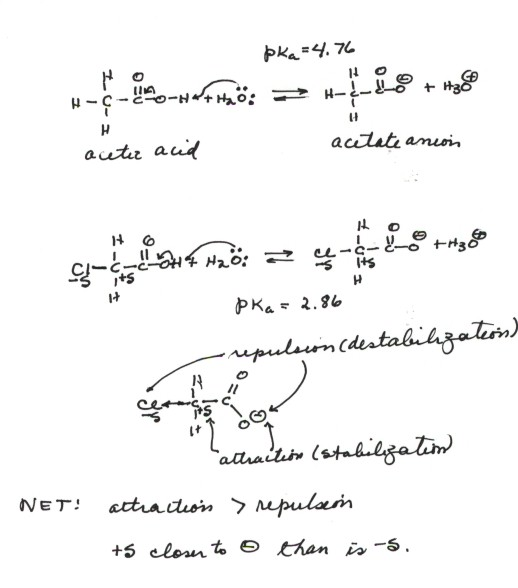## EQUILIBRIUM POSITION IN ACID/BASE REACTIONS

### THE QUALITATIVE CRITERION

• It is important to realize that if a B-L acid reacts with a B-L base, the result is the formation of a new B-L acid and a new B-L base. Thus, if H-A reacts with B-, the result is the formation of A- and H-B. Thus two B-L acids (HA and HB) are involved,one on the reactant side (the left hand side of the equation) and one on the product side (the right hand side of the equation).

### We can do much better than a simple qualitative criterion merely by using the pK values which we would need anyway for even a qualititative criterion.

• The simple rule is that the equilibrium constant for the given reaction of a Bronsted-Lowry acid with a general B-L base is the quotient of the Ka values of the acid on the reactant side divided by that of the acid on the product side. Be careful to remember that it is R/P, not the other way around. (This is not like writing an equilbrium constant in terms of concentrations).

### The concept of B-L acidity/basicity focuses on the proton, which is of course, a very important species in both inorganic and organic chemistry. More specifically, it focuses upon the transfer of a proton from one species to another, to form a new covalent bond. Professor G.N.Lewis broadened the concept of acidity/basicity so that covalent bond formation processes which do not involve the proton may be included in the same class. A B-L acid donates a proton (with no electrons) to a base which contributes both electrons to the resulting covalent bond. Lewis extended the concept of acidity/basicity by defining a Lewis acid as any chemical species which can form a covalent bond by accepting two electron from a base. A Lewis base, by the same token, is any species which can supply a pair of electrons to form a covalent bond. Any Bronsted-Lowry base is therefore also a Lewis base and conversely. All that is required is an available electron pair. In contrast, there are many Lewis acids which are not Bronsted acids, and indeed which may not contain an available proton at all.

• The reaction between ammonia (NH3) and BF3 is an excellent example. Ammonia is a B-L base and also a Lewis base, because it can supply an electron pair to form a covalent bond. But boron trifluoride is by no means a B-L acid, since it has no protons. Nevertheless, it reacts with ammonia in a fashion similar to a B-L acid, by forming a covalent bond, using the same electron pair of ammonia that a B-L acid would use. In this case the new bond is a B-N bond.